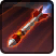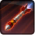# Taktischer SchlagConditions

## Used by

Used by
• Class 16141176590901306922

## Related effects, buffs and debuffs

Please click on an effect below to view its details.

• [hidden] [2s]Effect #1

 Slot: Buff Duration: 2 Sek. Tick rate: 0.5 Sek. # occurrences: 0
• On Apply
• [target override]

Only when the following conditions are met:

• <
• If PRIMARY TARGET is alive
AND
>

Perform the following actions:

• Add effect #2 to TARGET from TARGET
• When effect ticks the 1th time
• [target override]

Only when the following conditions are met:

• <
• If PRIMARY TARGET does not have any effect with the tag tag.​abl.​operation.​iokath.​boss.​izax.​izax.​missile_inbound_delay
AND
• If PRIMARY TARGET is a player
AND
• If PRIMARY TARGET is alive
AND
• Unknown (84)
- Negated = (bool) false
- Block Identifier = (int) 1
>

Perform the following actions:

• Add effect #3 to TARGET from TARGET
• When effect ticks the 1th time
• [target override]

Only when the following conditions are met:

• <
• If PRIMARY TARGET is a player
AND
• If PRIMARY TARGET is alive
AND
• Unknown (84)
- Negated = (bool) false
- Block Identifier = (int) 1
>

Perform the following actions:

• Add effect #3 to TARGET from TARGET
• When effect ticks the 2th time
• [target override]

Only when the following conditions are met:

• If difficulty is set to 16-man

Perform the following actions:

• Unknown (164)
- Ability Spec = (int) 16141020572542242042
- From Actor = (int) 3
- To Actor = (int) 3
• [hidden] [6s]Effect #2

 Slot: Buff Duration: 6 Sek. Tick rate: does not tick # occurrences: 0 Tags: tag.​abl.​operation.​iokath.​boss.​izax.​izax.​missile_inbound_delay Conditions: Can only by called by other effects
• On Apply

Perform the following actions:

• Unknown (183)
- Unknown (303) = (bool) true
- Unknown (306) = (bool) true
- Unknown (430) = (bool) false
- Unknown (310) = (string) aoePositionOptionType_Entity
- Unknown (311) = (string) aoeTarget_Ground
- Unknown (313) = (string) aoeScalarType_LiteralValue
- Unknown (317) = (string) aoeScalarType_LiteralValue
- Unknown (321) = (string) aoeScalarType_LiteralValue
- Unknown (325) = (string) aoeOrientationOptionType_WorldCoordinates
- Unknown (344) = (string) aoeSphere
- Unknown (349) = (string)
- Unknown (353) = (string) aoeScalarType_LiteralValue
- Unknown (354) = (string) aoeScalarType_LiteralValue
- Unknown (355) = (string) aoeScalarType_LiteralValue
- Unknown (367) = (string) aoeScalarType_LiteralValue
- Unknown (384) = (string)
- Unknown (386) = (string) aoeAbilityCaster_Caster
- Unknown (387) = (string) aoeAbilityTarget_Target
- Unknown (431) = (string) aoeRotation_None
- Ability Spec = (int) 16140923690223061629
- Unknown (307) = (int) 1
- Unknown (308) = (int) 1
- Tick Rate = (float) 5.4
- Unknown (304) = (float) 6
- Unknown (305) = (float) 6
- Unknown (314) = (float) 0
- Unknown (318) = (float) 0
- Unknown (322) = (float) 0
- Unknown (362) = (float) 0
- Unknown (363) = (float) 0
- Unknown (364) = (float) 0
- Unknown (368) = (float) 0.5
- Unknown (390) = (float) 0
- Unknown (398) = (float) 5.4
• Play appearance epp . operation . iokath . boss . izax . izax . missile_battery_projectile_left_01, dependent on calling effect
• [hidden] [7s]Effect 4169159113965938

 Slot: Buff Duration: 7 Sek. Tick rate: does not tick # occurrences: 0 Tags: tag.​abl.​operation.​iokath.​boss.​izax.​izax.​missile_inbound_delay Conditions: Can only by called by other effects
• On Apply

Perform the following actions:

• Unknown (183)
- Unknown (303) = (bool) true
- Unknown (306) = (bool) false
- Unknown (430) = (bool) false
- Unknown (310) = (string) aoePositionOptionType_Entity
- Unknown (311) = (string) aoeTarget_Ground
- Unknown (313) = (string) aoeScalarType_LiteralValue
- Unknown (317) = (string) aoeScalarType_LiteralValue
- Unknown (321) = (string) aoeScalarType_LiteralValue
- Unknown (325) = (string) aoeOrientationOptionType_EntityFacing
- Unknown (326) = (string) aoeTarget_Caster
- Unknown (344) = (string) aoeSphere
- Unknown (349) = (string)
- Unknown (353) = (string) aoeScalarType_LiteralValue
- Unknown (354) = (string) aoeScalarType_LiteralValue
- Unknown (355) = (string) aoeScalarType_LiteralValue
- Unknown (367) = (string) aoeScalarType_LiteralValue
- Unknown (384) = (string)
- Unknown (386) = (string) aoeAbilityCaster_Caster
- Unknown (387) = (string) aoeAbilityTarget_Target
- Unknown (431) = (string) aoeRotation_None
- Ability Spec = (int) 16140937166569220173
- Unknown (307) = (int) 1
- Unknown (308) = (int) 1
- Tick Rate = (float) 5.4
- Unknown (304) = (float) 6
- Unknown (305) = (float) 6
- Unknown (314) = (float) 0
- Unknown (318) = (float) 0
- Unknown (322) = (float) 0
- Unknown (362) = (float) 0
- Unknown (363) = (float) 0
- Unknown (364) = (float) 0
- Unknown (368) = (float) 0.5
- Unknown (390) = (float) 0
- Unknown (398) = (float) 5.4
• Play appearance epp . operation . iokath . boss . izax . izax . missile_battery_projectile_right_01, dependent on calling effect